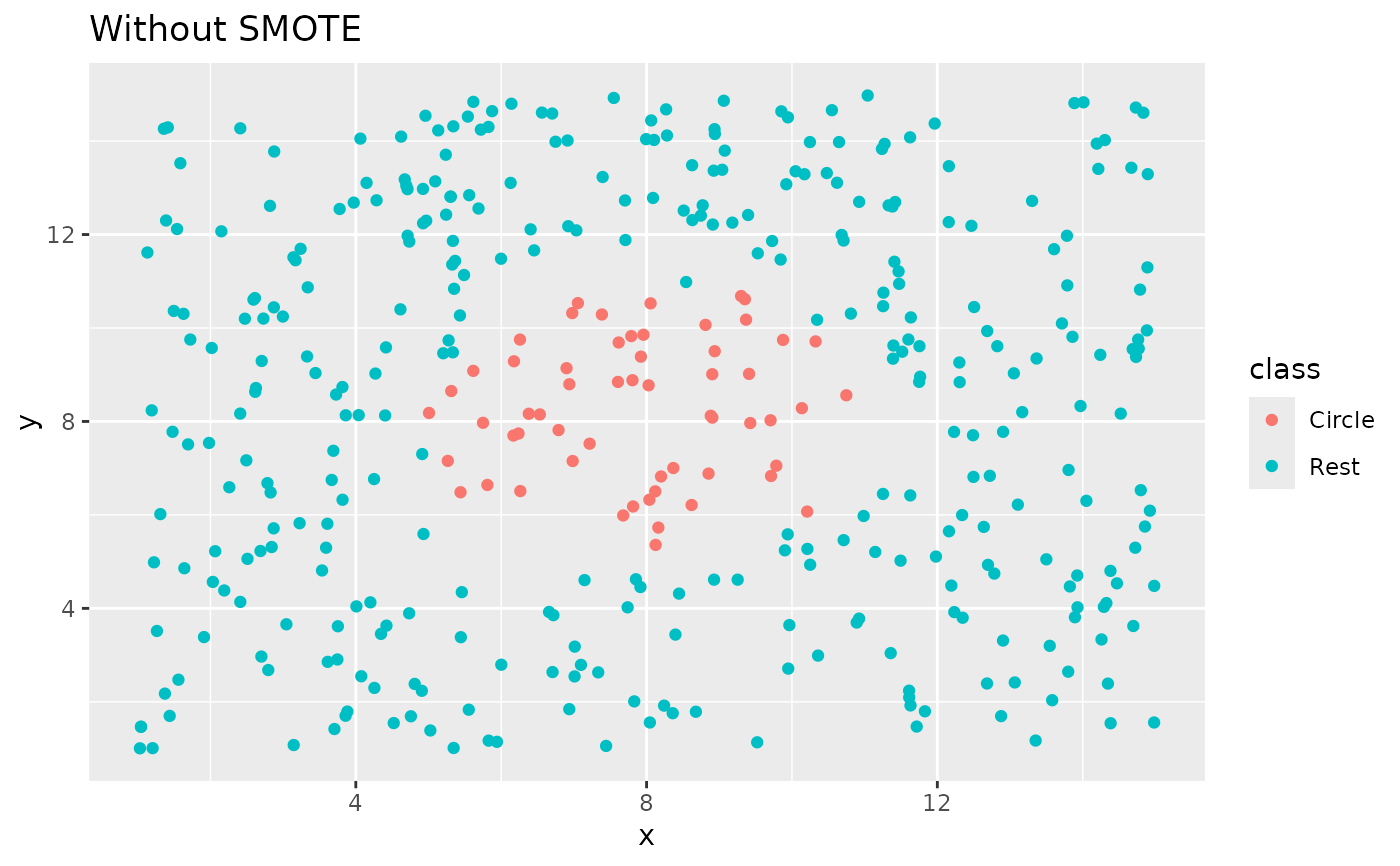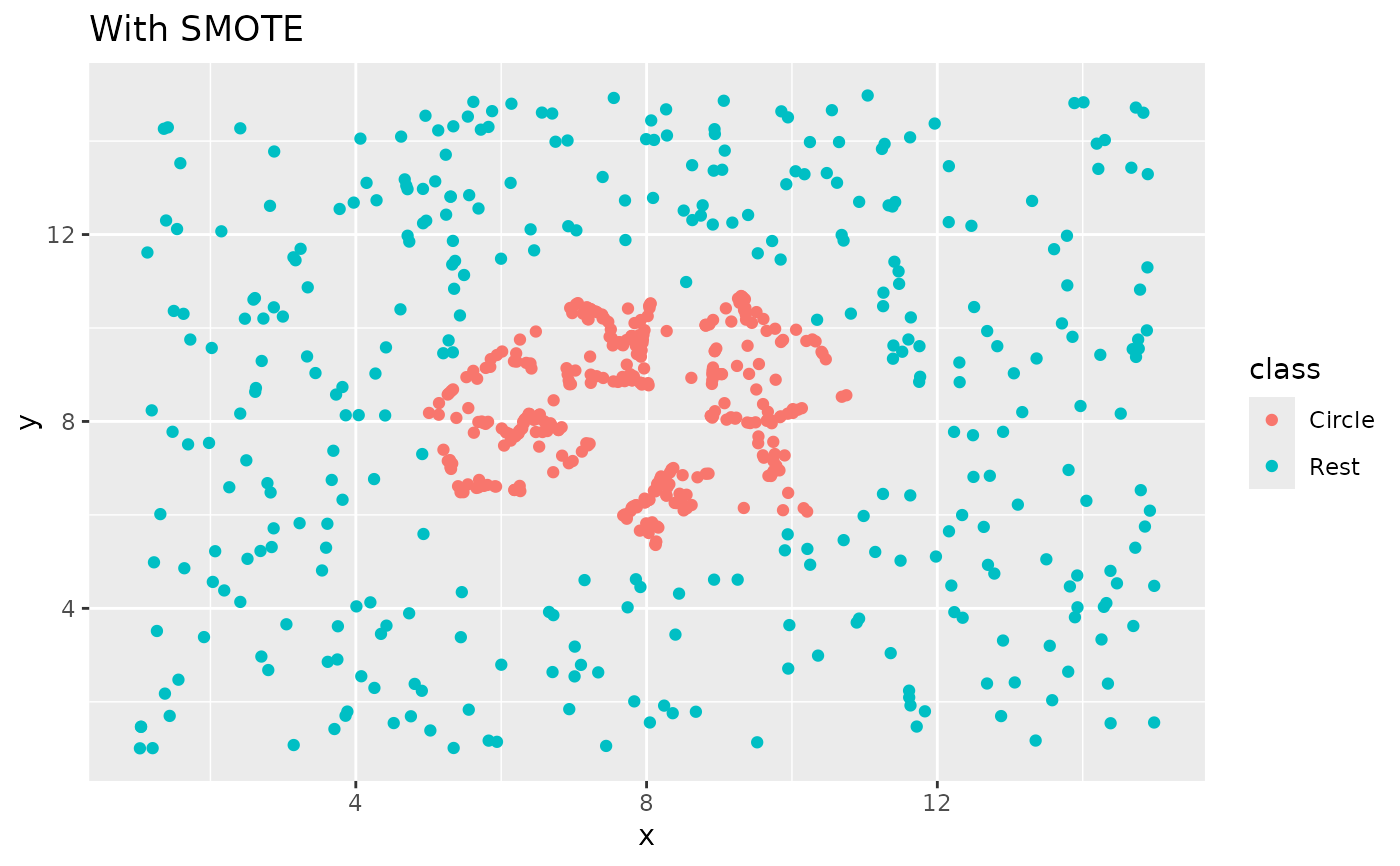step_smote creates a specification of a recipe step that generate new examples of the minority class using nearest neighbors of these cases.

step_smote(
recipe,
...,
role = NA,
trained = FALSE,
column = NULL,
over_ratio = 1,
neighbors = 5,
skip = TRUE,
seed = sample.int(10^5, 1),
id = rand_id("smote")
)

# S3 method for step_smote
tidy(x, ...)

## Arguments

recipe A recipe object. The step will be added to the sequence of operations for this recipe. One or more selector functions to choose which variable is used to sample the data. See selections() for more details. The selection should result in single factor variable. For the tidy method, these are not currently used. Not used by this step since no new variables are created. A logical to indicate if the quantities for preprocessing have been estimated. A character string of the variable name that will be populated (eventually) by the ... selectors. A numeric value for the ratio of the majority-to-minority frequencies. The default value (1) means that all other levels are sampled up to have the same frequency as the most occurring level. A value of 0.5 would mean that the minority levels will have (at most) (approximately) half as many rows than the majority level. An integer. Number of nearest neighbor that are used to generate the new examples of the minority class. A logical. Should the step be skipped when the recipe is baked by bake.recipe()? While all operations are baked when prep.recipe() is run, some operations may not be able to be conducted on new data (e.g. processing the outcome variable(s)). Care should be taken when using skip = TRUE as it may affect the computations for subsequent operations An integer that will be used as the seed when smote-ing. A character string that is unique to this step to identify it. A step_smote object.

## Value

An updated version of recipe with the new step added to the sequence of existing steps (if any). For the tidy method, a tibble with columns terms which is the variable used to sample.

## Details

The parameter neighbors controls the way the new examples are created. For each currently existing minority class example X new examples will be created (this is controlled by the parameter over_ratio as mentioned above). These examples will be generated by using the information from the neighbors nearest neighbor of each example of the minority class. The parameter neighbors controls how many of these neighbor are used.

All columns in the data are sampled and returned by juice() and bake().

All columns used in this step must be numeric with no missing data.

When used in modeling, users should strongly consider using the option skip = TRUE so that the extra sampling is not conducted outside of the training set.

Chawla, N. V., Bowyer, K. W., Hall, L. O., and Kegelmeyer, W. P. (2002). Smote: Synthetic minority over-sampling technique. Journal of Artificial Intelligence Research, 16:321-357.

## Examples

library(recipes)
library(modeldata)
data(credit_data)

sort(table(credit_data$Status, useNA = "always")) #> #> <NA> bad good #> 0 1254 3200 ds_rec <- recipe(Status ~ Age + Income + Assets, data = credit_data) %>% step_meanimpute(all_predictors()) %>% step_smote(Status) %>% prep() sort(table(bake(ds_rec, new_data = NULL)$Status, useNA = "always"))
#>
#>    0 3200 3200
# since skip defaults to TRUE, baking the step has no effect
baked_okc <- bake(ds_rec, new_data = credit_data)
table(baked_okc$Status, useNA = "always") #> #> bad good <NA> #> 1254 3200 0 ds_rec2 <- recipe(Status ~ Age + Income + Assets, data = credit_data) %>% step_meanimpute(all_predictors()) %>% step_smote(Status, over_ratio = 0.2) %>% prep() table(bake(ds_rec2, new_data = NULL)$Status, useNA = "always")
#>
#> 1254 3200    0
library(ggplot2)

ggplot(circle_example, aes(x, y, color = class)) +
geom_point() +
labs(title = "Without SMOTE")recipe(class ~ ., data = circle_example) %>%
step_smote(class) %>%
prep() %>%
bake(new_data = NULL) %>%
ggplot(aes(x, y, color = class)) +
geom_point() +
labs(title = "With SMOTE")